Courses

# Test: Reaction Intermediate Level - 1

## 30 Questions MCQ Test Organic Chemistry | Test: Reaction Intermediate Level - 1

Description
This mock test of Test: Reaction Intermediate Level - 1 for Chemistry helps you for every Chemistry entrance exam. This contains 30 Multiple Choice Questions for Chemistry Test: Reaction Intermediate Level - 1 (mcq) to study with solutions a complete question bank. The solved questions answers in this Test: Reaction Intermediate Level - 1 quiz give you a good mix of easy questions and tough questions. Chemistry students definitely take this Test: Reaction Intermediate Level - 1 exercise for a better result in the exam. You can find other Test: Reaction Intermediate Level - 1 extra questions, long questions & short questions for Chemistry on EduRev as well by searching above.
QUESTION: 1

### Which of the following is most reactive as a nucleophile?

Solution:

The most nucleophile is dependent on the compound which has a tendency to give an electron. In the compound there is oxygen and one has sulphur. The sulphur has big in size that why it has more tendency to donate an electron. The correct answer is PhS-

QUESTION: 2

Solution:
QUESTION: 3

### The relative acidity of the indicated H in each of the following is: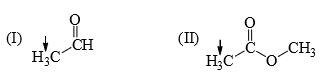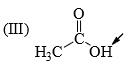Solution:
QUESTION: 4

The relative reactivity towards reaction with MeMgBr of the following is: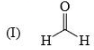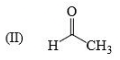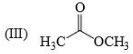Solution:

Order of reactivity: Aldehyde> Ketone> Esters.

QUESTION: 5

The relative nucleophilicity in polar, protic, solvents of the following is: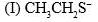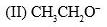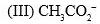Solution:

Explanation : In a polar protic solvent (CH3OH), nucleophilicity increases down a column of the periodic table. So S- is more nucleophilic than O-.

For two species with the same attacking atom, the more basic is the more nucleophilic, so CH3CH2O- is more nucleophilic than CH3CO2-

CO2- give identical str resonance hybrids more solvated, very less nucleophilic.

QUESTION: 6

The acidity of the protons H in each of the following is: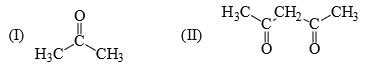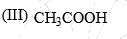Solution:
QUESTION: 7

The relative nucleophilicity in polar, protic, solvents of the following is:
(I) CH3OH                  (II) CH3SH                (III) CH3NH2

Solution:
QUESTION: 8

Reactivity of the following towards reaction with NaBH4 is: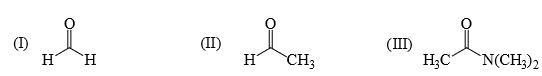Solution:
QUESTION: 9

Rate of reaction of CH3COCl/AlCl3 with each of the following is: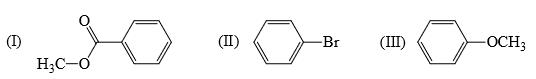Solution:
QUESTION: 10

Identify correct C—O bond length order: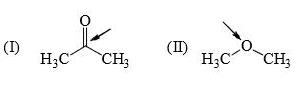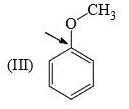Solution:

I is the shortest because of double bond.

III is next because of resonance.

II is last as there is no kind of bond strengthening.

Hence B is correct.

QUESTION: 11

The resonance energy of each of the following is: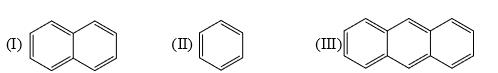Solution:

Explanation : Resonance energy per ring decreases as ring size increases

So, III > I > II (Resonance energy/ring)

Anthracene>Benzene>Naphthalene.

QUESTION: 12

Identify order of per ring resonance energies of each of the following: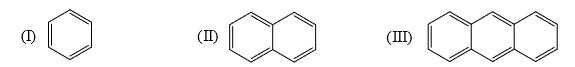Solution:
QUESTION: 13

The relative stability of the following carbocations is: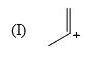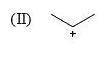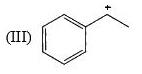Solution:

Explanation : The dispersal of the charge stabilizes the carbocation. More the number of alkyl groups, the greater the dispersal of positive charge and therefore, more the stability of carbocation,is an electron donating group, thus it will increases the stability of carbocation, hence the expected order is, (iii)>(ii)>(i).

QUESTION: 14

Which of the following systems are resonance contributions of the radical shown below: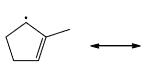Solution: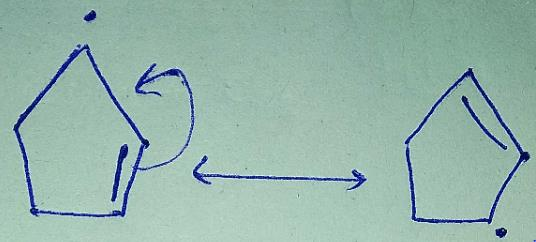Since, a free radical does the homolysis of pi bond.

QUESTION: 15

Imidazole has a pKa = 7 with respect to its conjugate acid. Which N is protonated in this conjugate acid and why?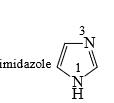Solution:
QUESTION: 16

Cyclopentadiene has a pKa = 15, whereas cyclopentane has a pKa > 50. This is because:

Solution:

Since after deprotonation cyclopentadiene becomes stable by aromaticity, So, it will definitely have a higher acidic strength than that of cyclopentane and consequently lower pKa value.

Further, no such stability factor has a role in the case of cyclopentane so it has lower acidic strength comparatively.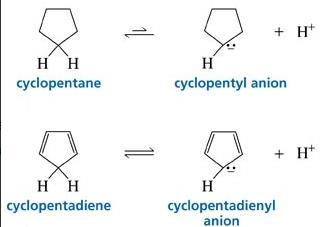QUESTION: 17

Which of the following is most likely to undergo a favourable hydride shift?

Solution:
QUESTION: 18

Arrange the following intermediate into decreasing order of stability.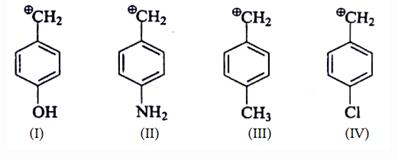Solution: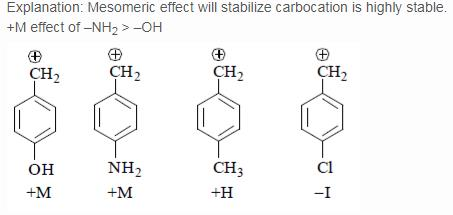QUESTION: 19

Which of the following forms most stable carbocation upon removal of OH–:

Solution:
QUESTION: 20

Which of the following carbonium ion is most stable?

Solution:

A is the correct option as 9- alpha Hydrogens are present. More alpha hydrogen, more hyperconjugation, more stability.

QUESTION: 21

Which of the following carbocations would not likely rearrange to a more stable carbocation?

Solution:
QUESTION: 22

Which carbocation is the most stabilized:

Solution:
QUESTION: 23

Which one of the following carbocation would you expect to rearrange?

Solution:

In option B and C, after carbocation rearrangement, it will remain 2°, therefore, rearrangement will not take place.
In option D, after carbocation rearrangement 3° will become 2° or 1°, therefore, rearrangement will not take place.
In option A, after rearrangement, carbocation will become 3° from 2°, therefore, this rearrangment will take place.

QUESTION: 24

Which carbocation is the most stable?

Solution:
QUESTION: 25

Arrange stability of the given carbocations in decreasing order: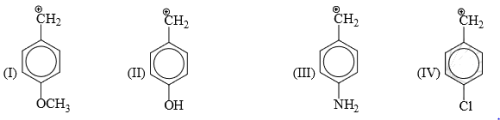Solution:

Explanation : The carbocation here is stabilized when there is an electron-donating group present.

More the activating effect of the group, more stable is the cation. Out of given options, I is most stable due to ability of oxygen to activate the ring with its lone pair of electrons.

Out of III and I,III is more stable as the lone pair of nitrogen in II is less available due to Ac group. IV is least stable due to electron- withdrawing effect of Cl atom.

The correct answer is : IV < II < III < I

QUESTION: 26

Which intermediate is involved in the reaction given below?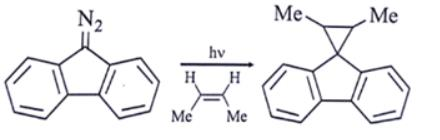Solution:

Ans: d

Explanation: As we can see, the first step in the presence of light carbene is formed.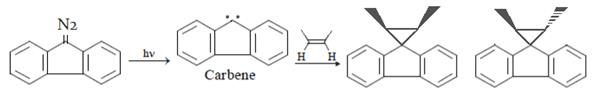QUESTION: 27

Consider the following statements: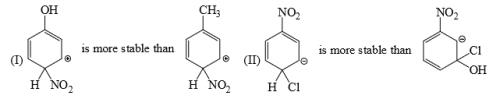Solution:
QUESTION: 28

Which is the most stable arenium carbocation:

Solution:
QUESTION: 29

Which of the following statements is correct: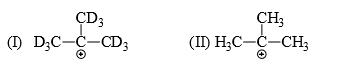Solution:
QUESTION: 30

List the following carbocations in order of decreasing stability (starting with the most stable):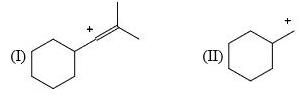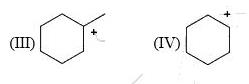Solution:

Explanation : I have an sp2 hybridized carbocation which is highly unstable. Hence, I will be the least stable of all. III will be the most stable as it is tertiary carbocation.

Among II and IV, IV has 4 alpha− hydrogens whereas II has only, α− hydrogens. Also IV is a secondary carbocation while II is a primary one. Hence IV will be more stable than II.

Hence, the decreasing order of stability or stabilization energy is III>IV>II>I

Track your progress, build streaks, highlight & save important lessons and more!

### Similar Content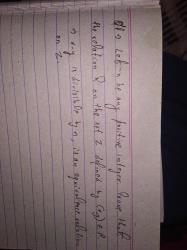### Related tests